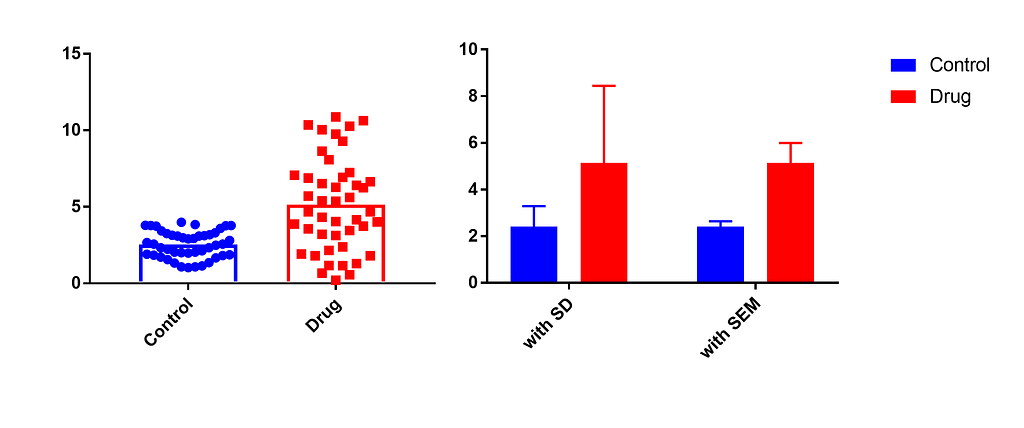# Understanding Context: Experimental Biology and Statistics

Coming from a Maths background, Statistics has always been part of my coursework. But it was always a bit hard for me to understand the theory without applying it. Now, here at a metabolism lab while using those concepts I thought it might be a good idea to revisit the theory with a given context. I wanted to share my notes with those who find it helpful.

Bar Graphs and Error Bars:

I have seen my share of bar graphs over the years. But somehow I was used to seeing standard deviation as error bars and here, standard error of mean seems to be the norm. Let me try to explain what the difference is and why the latter could be a better choice in experimental biology.

Standard Deviation (SD): It is a measure of the variability of data points with respect to the mean. For sample standard deviation (s), the mean is sample mean (x̅).

s = √(Σ(x- x̅)²/n-1)

Standard Error of Mean (SEM): It is the standard deviation of the sampling distribution of the sample mean. Here σ is population standard deviation.

σx̅ = σ/√n

As the definition of SD says, it tells you something about the sampled data, it gives the spread of your sample. What SEM tells you is something about the precision of population mean. This is true for any population as long as the sample size is large enough (n≥30) by Central Limit Theorem, because then the sample distribution of sample mean approaches a normal distribution with its mean equal to mean of original population. As we seldom know the value of σ, we use s, which approaches σ as our sample size increases.

In experimental biology, SEM is helpful because the hypotheses often involve comparing two conditions. For example, if one wants to study the effects of a drug on the weight of mice, they would want to compare the mice with drug and without drug (generally referred to as control). The variable of interest is the weight of mice and the two populations are : weights of all mice in the world with drug and weights of all mice without drug. Experiments are done with a certain number of mice from this population and results are plotted.Image on the left: Data points in two conditions, right: Bar graphs for the earlier data points with different error bars

In the above plots, while the SD just tells variation of data in individual samples, SEM compares the means of both populations (we can visually compare the means of both populations by the distance in error bars) and also tells about the variations in both samples if the sample size is same.

Assumption of Normality:

Biological parameters like weight, height, blood volume, etc. are often assumed to follow normal distribution. The reasoning behind this is that any biological parameter is an aggregation of a large number of random events and therefore, by Central Limit Theorem (CLT), follows normal distribution. This assumption helps when the number of samples collected is smaller, which is often the case in experimental biology. By a rule of thumb, if n ≤30 and the population is normally distributed, the sampling distribution of sample mean follows a distribution known as Student’s t-distribution. Now, using this information we can compare the effects of drug on the mean of the biological parameter using t-test.

Caveats:

With assumptions comes responsibility! When studying a graph in a research paper, it is important to check what the error bar represents, and if it is SEM, n has to be taken into account. With n ≤3 , it might be a good idea to plot the points themselves. One should keep in mind that n is the number of biological replicates (different mice from the mice population) and not technical replicates (samples from the same mouse) because the latter won’t be representative of original population. Lots of people have questions about the normality assumption and it might be a good idea to understand your population more before publishing results. Well, reproducible results are the best results, so maybe repeating the experiments is the way to go before saying things conclusively.